Name: ___________________Date:___________________

 Email us to get an instant 20% discount on highly effective K-12 Math & English kwizNET Programs!

### Grade 4 - Mathematics8.9 Types of Fractions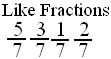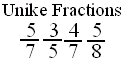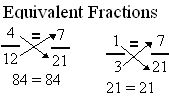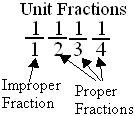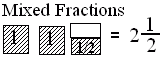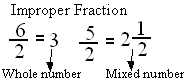Directions: Answer the following questions. Also write at least 5 examples of each type of fractions.Name: ___________________Date:___________________

### Grade 4 - Mathematics8.9 Types of Fractions

 Q 1: A fraction less than 1 is called a proper fraction. A fraction equal to one and more than 1 is called _________.proper fractionimproper fractionmixed fraction Q 2: Fractions with same denominators are calledlike fractionsunlike fractions Q 3: When a products obtained by cross multiplication give the same number, then the fractions are ____(or equal).mixedproperequivalent Q 4: If the numerator is less than the denominator in a fraction, the fraction is called a _______.proper fractionimproper fractionmixed fraction Q 5: Fraction with numerator 1 is called a unit fraction. Are all unit fractions proper?YesNo Q 6: An improper fraction is sometimes a mixed number or sometimes a whole number.FalseTrue Q 7: If the numerator is more than the denominator in a fraction, the fraction is called a _________.mixed fractionimproper fractionproper fraction Q 8: Fractions with different denominators are calledunlike fractionslike fractions Question 9: This question is available to subscribers only! Question 10: This question is available to subscribers only!

#### Subscription to kwizNET Learning System costs less than \$1 per month & offers the following benefits:

• Unrestricted access to grade appropriate lessons, quizzes, & printable worksheets
• Instant scoring of online quizzes
• Progress tracking and award certificates to keep your student motivated
• Unlimited practice with auto-generated 'WIZ MATH' quizzes